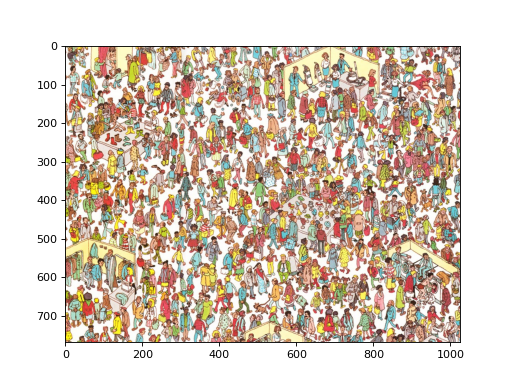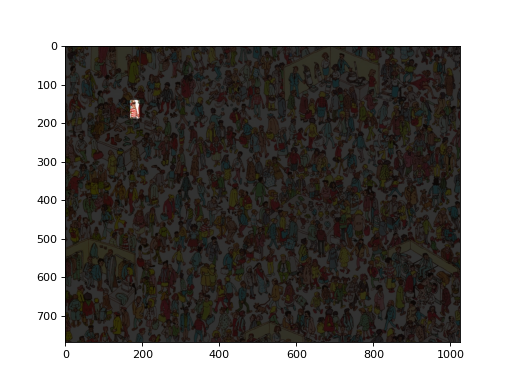# Finding Wally¶

This was originally an answer on stackoverflow We can use it as a simple tutorial example.

The problem is to find Wally (who goes by Waldo in the US) in the following image:

```from pylab import imshow, show
import mahotas
import mahotas.demos
imshow(wally)
show()
```From October 11 2013 onwards (version 1.0.4 or later), you can get the Wally image from mahotas as:

```import mahotas.demos
```

Can you see him?

```wfloat = wally.astype(float)
r,g,b = wfloat.transpose((2,0,1))
```

Split into red, green, and blue channels. It’s better to use floating point arithmetic below, so we convert at the top.

```w = wfloat.mean(2)
```

w is the white channel.

```pattern = np.ones((24,16), float)
for i in xrange(2):
pattern[i::4] = -1
```

Build up a pattern of +1,+1,-1,-1 on the vertical axis. This is Wally’s shirt.

```v = mahotas.convolve(r-w, pattern)
```

Convolve with red minus white. This will give a strong response where the shirt is.

```mask = (v == v.max())
```

Look for the maximum value and dilate it to make it visible. Now, we tone down the whole image, except the region or interest:

```wally -= .8*wally * ~mask[:,:,None]
```

And we get the following:

```wfloat = wally.astype(float)
r,g,b = wfloat.transpose((2,0,1))
w = wfloat.mean(2)
pattern = np.ones((24,16), float)
for i in xrange(2):
pattern[i::4] = -1
v = mahotas.convolve(r-w, pattern)
mask = (v == v.max())